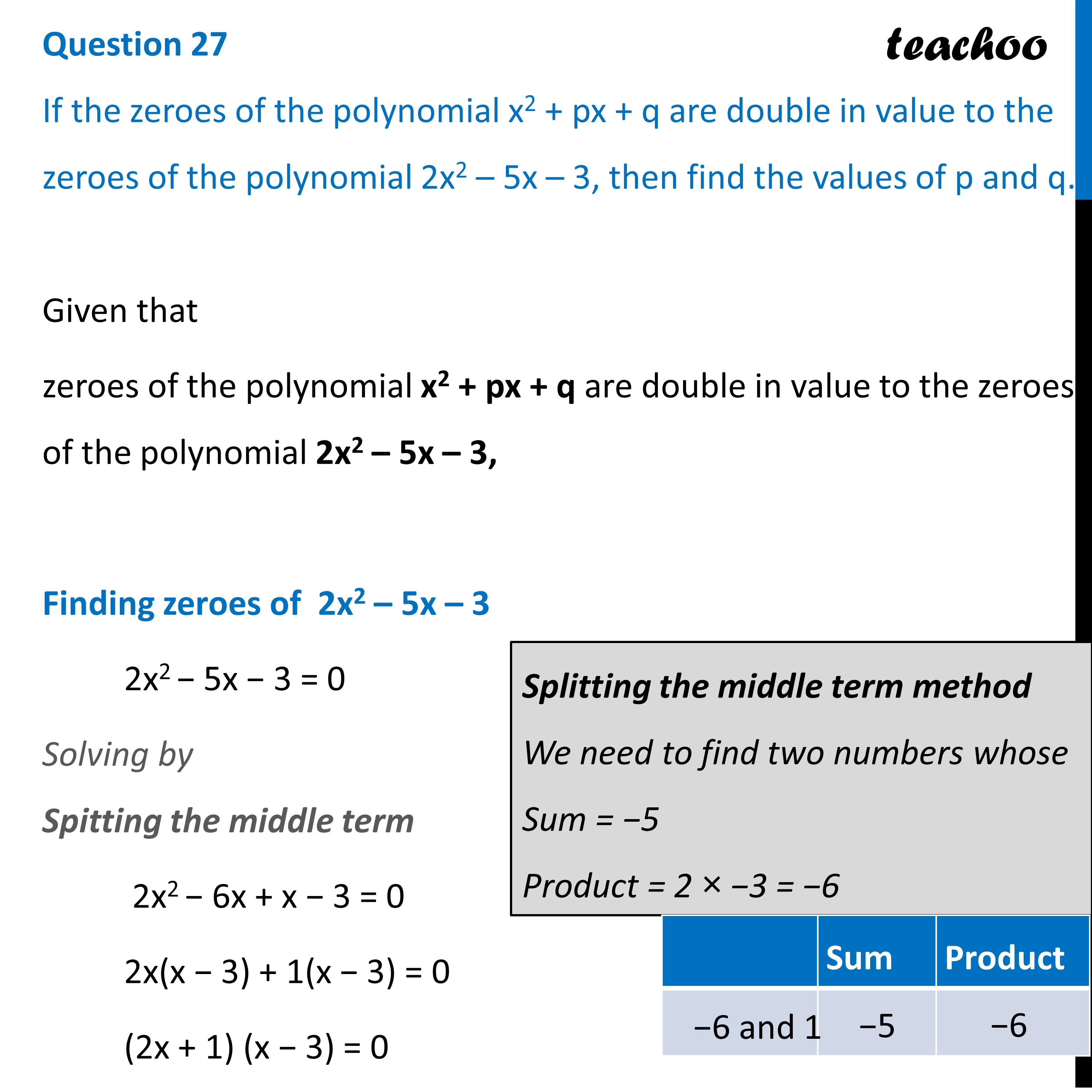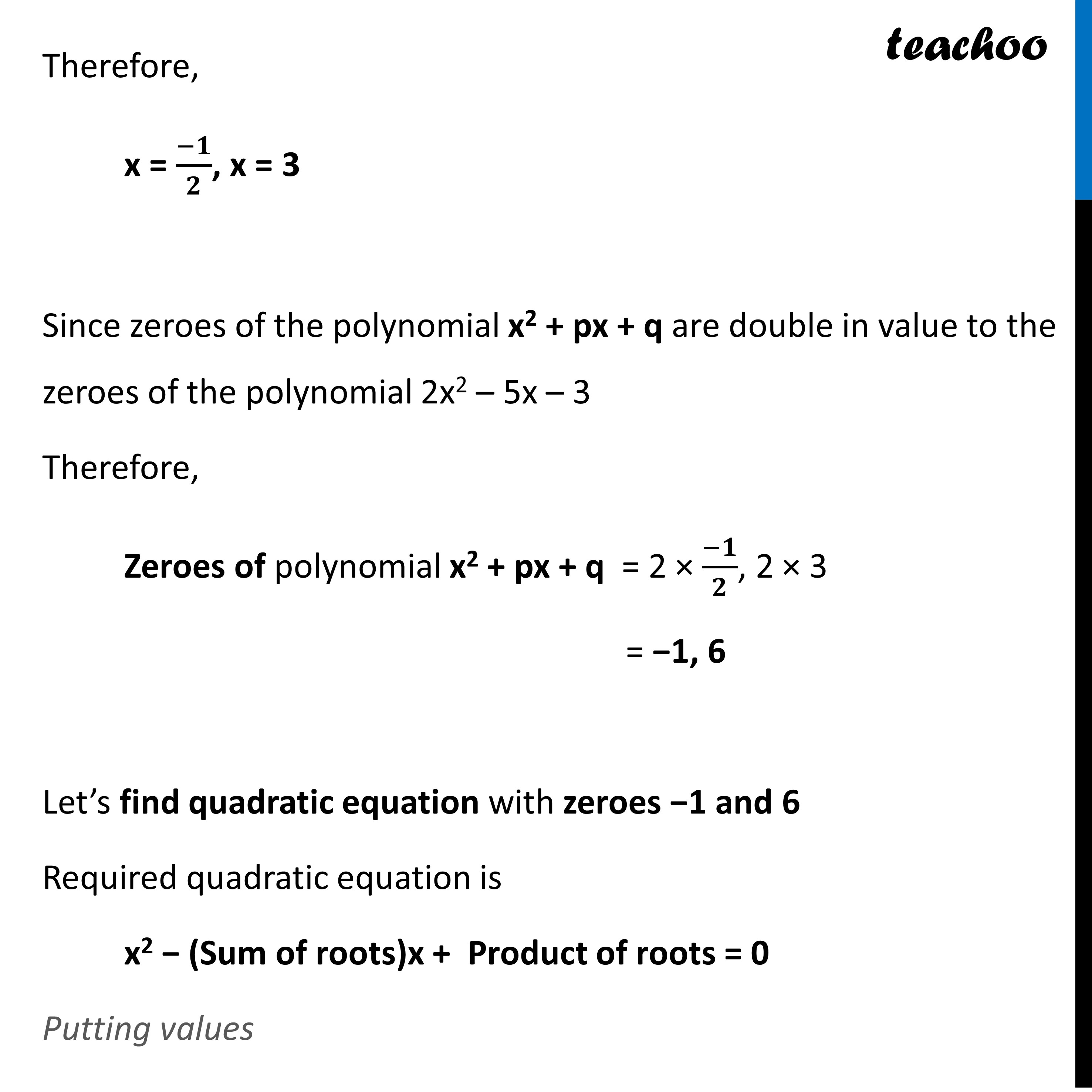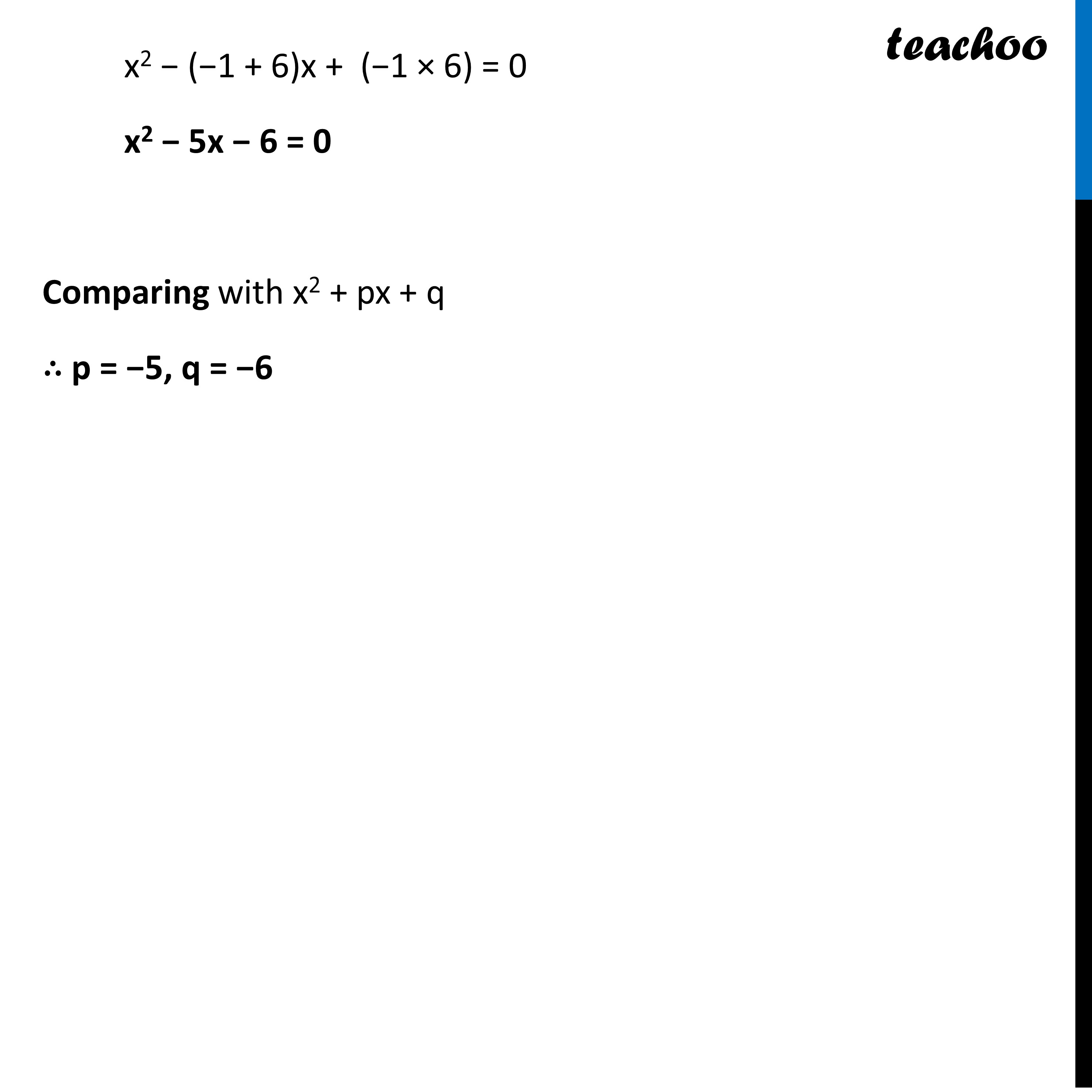CBSE Class 10 Sample Paper for 2023 Boards - Maths Standard

Class 10
Solutions of Sample Papers for Class 10 Boards

## If the zeroes of the polynomial x 2 + px +q are double in value to the zeroes of the polynomial 2x 2 – 5x – 3, then find the values of p and q.Learn in your speed, with individual attention - Teachoo Maths 1-on-1 Class

### Transcript

Question 27 If the zeroes of the polynomial x2 + px + q are double in value to the zeroes of the polynomial 2x2 – 5x – 3, then find the values of p and q.Given that zeroes of the polynomial x2 + px + q are double in value to the zeroes of the polynomial 2x2 – 5x – 3, Finding zeroes of 2x2 – 5x – 3 2x2 − 5x − 3 = 0 Solving by Spitting the middle term 2x2 − 6x + x − 3 = 0 2x(x − 3) + 1(x − 3) = 0 (2x + 1) (x − 3) = 0 Splitting the middle term method We need to find two numbers whose Sum = −5 Product = 2 × −3 = −6 Therefore, x = (−𝟏)/𝟐, x = 3 Since zeroes of the polynomial x2 + px + q are double in value to the zeroes of the polynomial 2x2 – 5x – 3 Therefore, Zeroes of polynomial x2 + px + q = 2 × (−𝟏)/𝟐, 2 × 3 = −1, 6 Let’s find quadratic equation with zeroes −1 and 6 Required quadratic equation is x2 − (Sum of roots)x + Product of roots = 0 Putting values x2 − (−1 + 6)x + (−1 × 6) = 0 x2 − 5x − 6 = 0 Comparing with x2 + px + q ∴ p = −5, q = −6